CBSE Class 12 Sample Paper for 2021 Boards

Class 12
Solutions of Sample Papers and Past Year Papers - for Class 12 Boards

## Find the equation of the normal to the curve y = 𝑥 + 1/x,   x > 0 perpendicular to the line 3𝑥 − 4𝑦 = 7.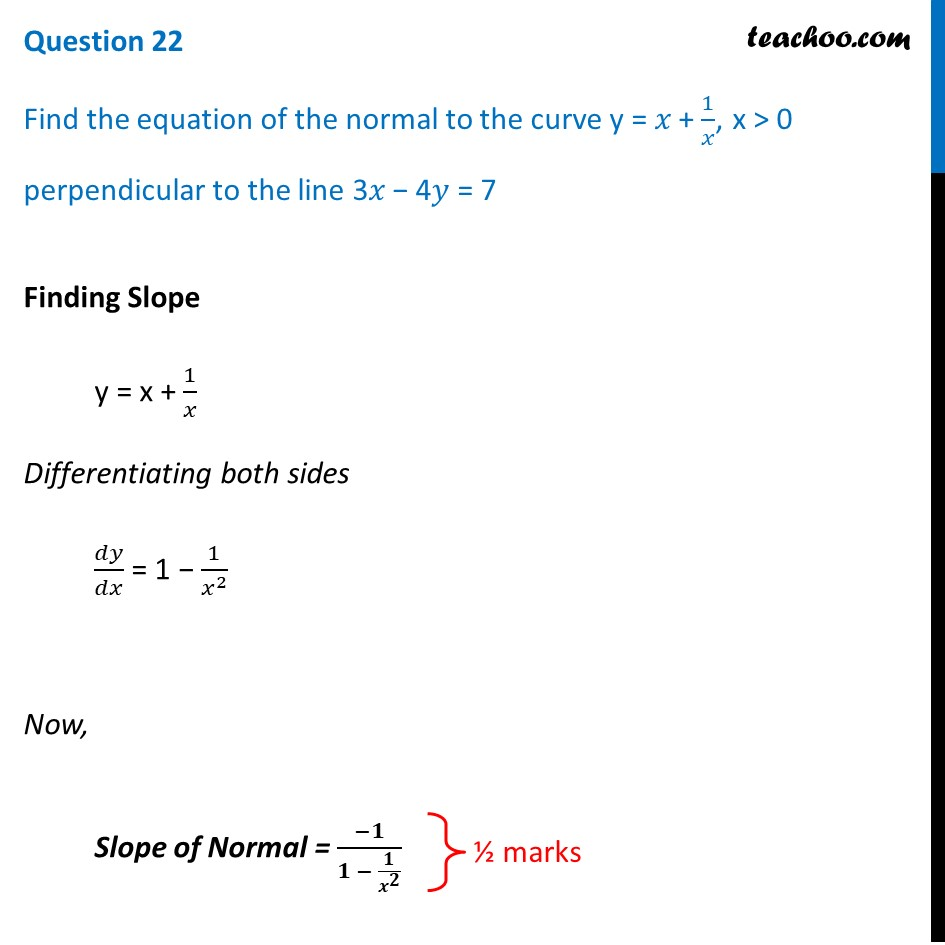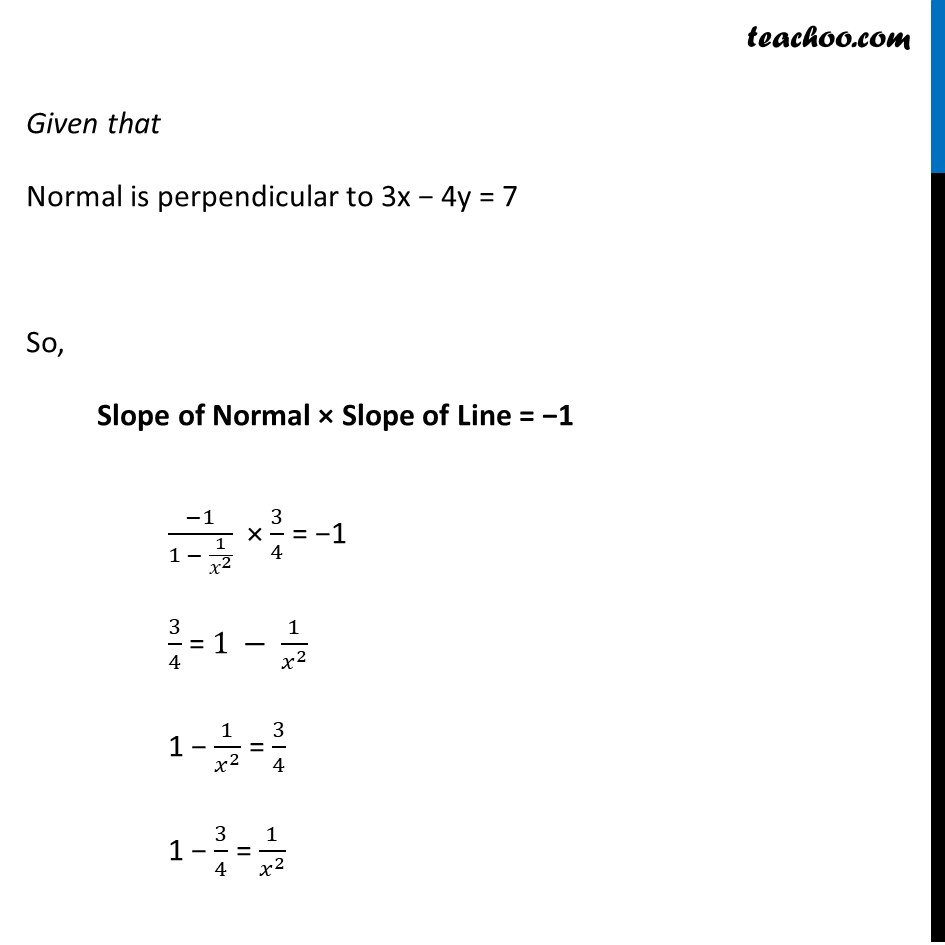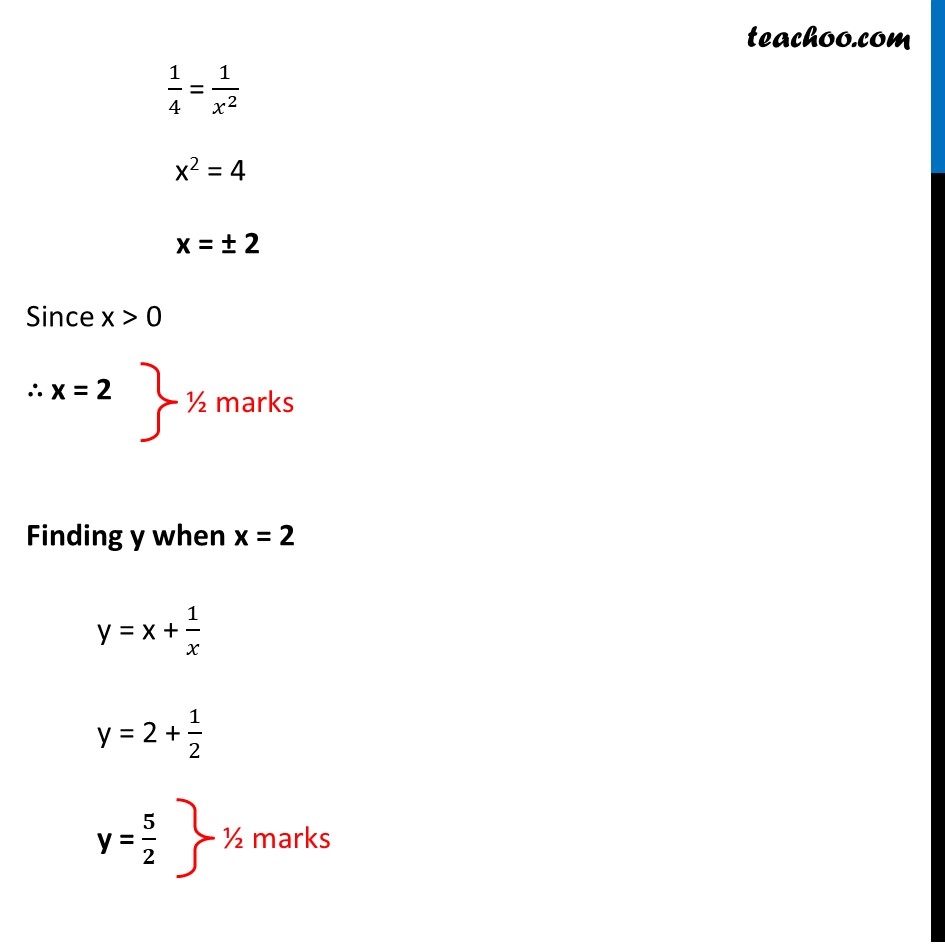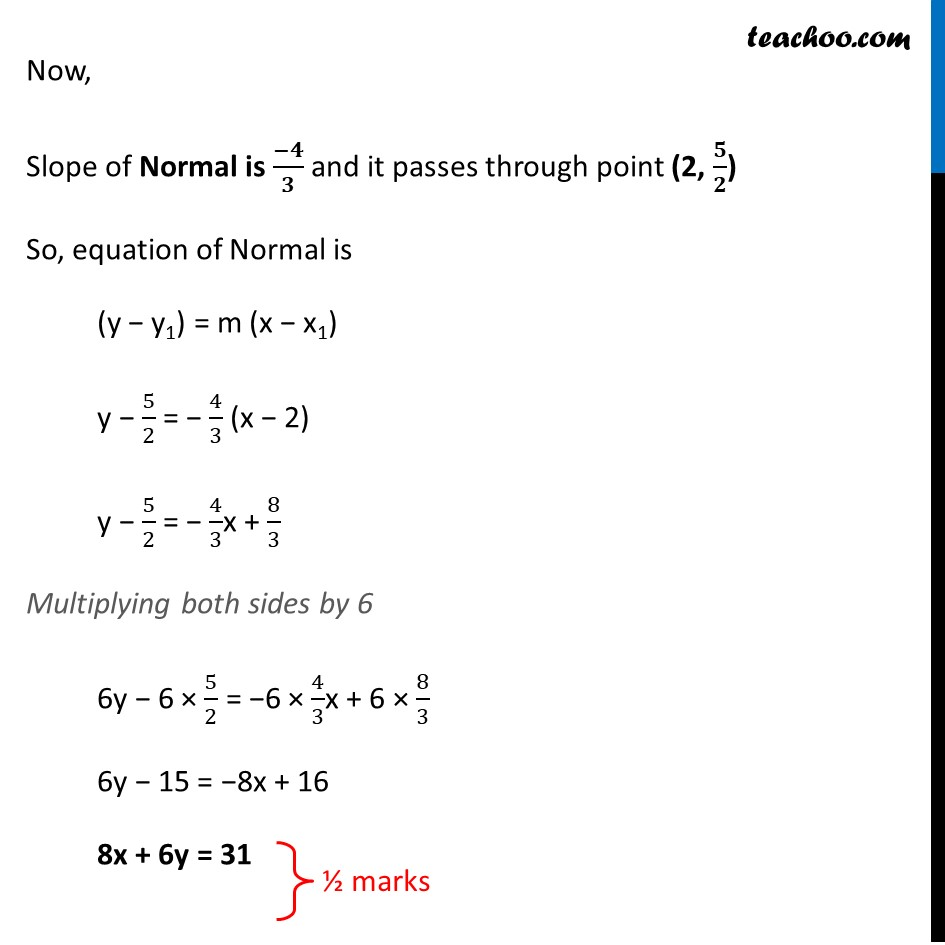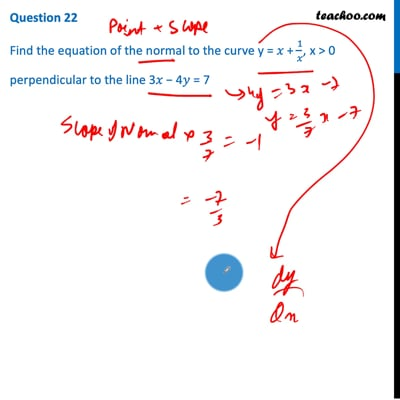This video is only available for Teachoo black users

Introducing your new favourite teacher - Teachoo Black, at only ₹83 per month

### Transcript

Question 22 Find the equation of the normal to the curve y = 𝑥 + 1/𝑥, x > 0 perpendicular to the line 3𝑥 − 4𝑦 = 7 Finding Slope y = x + 1/𝑥 Differentiating both sides 𝑑𝑦/𝑑𝑥 = 1 − 1/𝑥^2 Now, Slope of Normal = (−𝟏)/(𝟏 − 𝟏/𝒙^𝟐 ) Given that Normal is perpendicular to 3x − 4y = 7 So, Slope of Normal × Slope of Line = −1 (−1)/(1 − 1/𝑥^2 ) × 3/4 = −1 3/4 = 1 − 1/𝑥^2 1 − 1/𝑥^2 = 3/4 1 − 3/4 = 1/𝑥^2 1/4 = 1/𝑥^2 x2 = 4 x = ± 2 Since x > 0 ∴ x = 2 Finding y when x = 2 y = x + 1/𝑥 y = 2 + 1/2 y = 𝟓/𝟐 Now, Slope of Normal is (−𝟒)/𝟑 and it passes through point (2, 𝟓/𝟐) So, equation of Normal is (y − y1) = m (x − x1) y − 5/2 = − 4/3 (x − 2) y − 5/2 = − 4/3x + 8/3 Multiplying both sides by 6 6y − 6 × 5/2 = −6 × 4/3x + 6 × 8/3 6y − 15 = −8x + 16 8x + 6y = 31